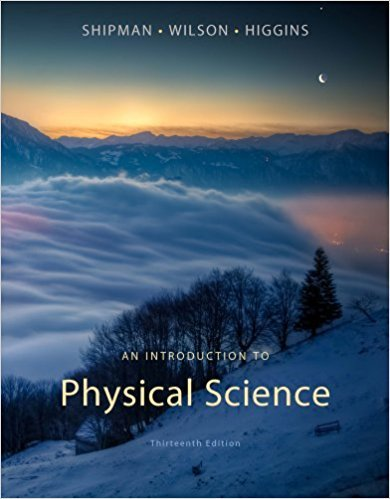×
×

# Which type of air mass is mainly responsible for mostlyISBN: 9781133109099 229

## Solution for problem 4 Chapter 20

An Introduction to Physical Science | 13th Edition

• Textbook Solutions
• 2901 Step-by-step solutions solved by professors and subject experts
• Get 24/7 help from StudySoup virtual teaching assistantsAn Introduction to Physical Science | 13th Edition

4 5 1 294 Reviews
14
3
Problem 4

Which type of air mass is mainly responsible for mostly warmweather in the conterminous United States? (20.2)(a) mP (b) cA(c) mT (d) cT

Step-by-Step Solution:
Step 1 of 3

Name _________________________________ Period _____ Coulomb's Law Practice Problems 1. Suppose that two point charges, each with a charge of +1 Coulomb are separated by a distance of 1 meter. (a) Will they attract or repel _______________(b) Determine the magnitude of the electrical force...

Step 2 of 3

Step 3 of 3

##### ISBN: 9781133109099

The answer to “Which type of air mass is mainly responsible for mostly warmweather in the conterminous United States? (20.2)(a) mP (b) cA(c) mT (d) cT” is broken down into a number of easy to follow steps, and 23 words. The full step-by-step solution to problem: 4 from chapter: 20 was answered by , our top Physics solution expert on 01/09/18, 07:57PM. This textbook survival guide was created for the textbook: An Introduction to Physical Science, edition: 13. An Introduction to Physical Science was written by and is associated to the ISBN: 9781133109099. This full solution covers the following key subjects: . This expansive textbook survival guide covers 24 chapters, and 736 solutions. Since the solution to 4 from 20 chapter was answered, more than 233 students have viewed the full step-by-step answer.

Unlock Textbook Solution